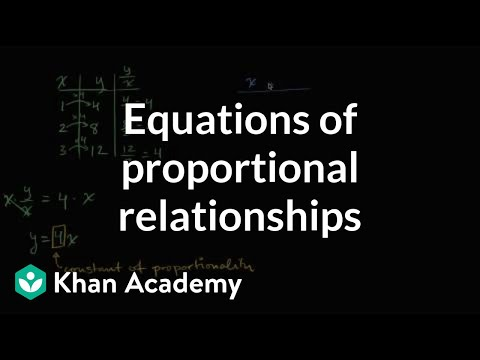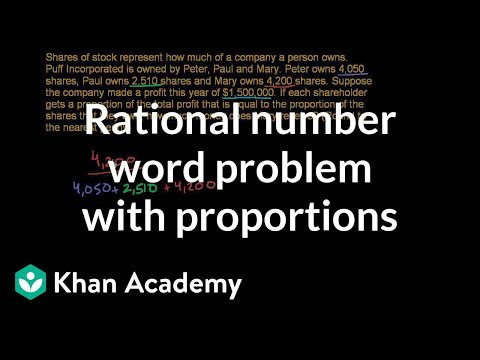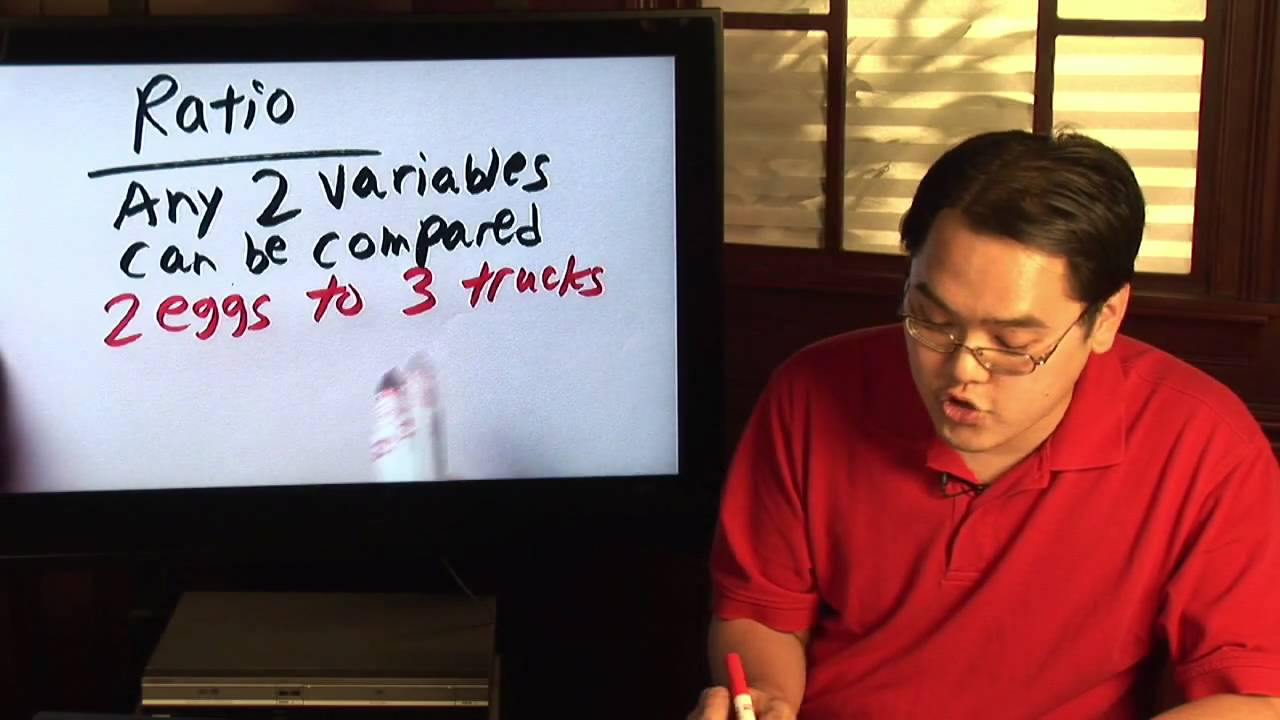# gratuity and commission - 7.RP.A.3

##By Geoff Olson

gratuity and commission# Writing proportional equations

##By Khan Academy

Learn how to write a proportional equation y=kx where k is the so-called constant of proportionality.# Identifying proportional relationships

##By Khan Academy

Is the volume of a square proportional to the side length of the square?# Identifying proportional relationships

##By Khan Academy

A proportionality problem about eating bananas# Identifying proportional relationships

##By Khan Academy

Given a table of ratios, watch as we test them for equivalence and determine whether the relationship is proportional.# Identifying proportional relationships

##By Khan Academy

The relationship between two variables is proportional ifï¿½ï¿½ï¿½ï¿½ï¿½ï¿½ï¿½ï¿½ï¿½ï¿½ï¿½ï¿½# Identifying proportional relationships

##By Khan Academy

Is the perimeter of a square proportional to the side length of the square?# Identifying proportional relationships with graphs

##By Khan Academy

Learn how to tell proportional relationships by drawing graphs. Key idea: the graph of a proportional relationship is a straight line through the origin.# Writing proportions

##By Khan Academy

Some examples of writing two ratios and setting them equal to each other to solve proportion word problems# Solving proportions

##By Khan Academy

Here's a great video where we explain the reasoning behind solving proportions. We'll put some algebra to work to get our answers, too.# Significance of points on graphs of proportions

##By Khan Academy

Graphical representation# Interpreting graphs of proportional relationships

##By Khan Academy

Learn how to read and understand graphs of proportional relationships# Represent proportions by equations

##By Khan Academy

Proportional relationships# DEPRECATED Rational number word problems

##By Khan Academy

Use your knowledge of proportions and fractions to determine shareholders profit in this word problem.# Fractions & Proportions : Understanding Math Ratios

##By

In math, ratio is a fraction in which two variables can compared. Discover how to convert ratios into fractions with help from a math teacher in this free vi...# Solving an Equation Involving Rational Exponents - Example 3

##By PatrickJMT

YouTube presents Solving an Equation Involving Rational Exponents - Example 3, an educational video resource on math.# Rate problems with fractions

##By Khan Academy

Determining rates with fractions# Triangles

##By MathPlanetVideos

Find the value of x in the triangle# Writing proportional equations

##By Khan Academy

Learn how to write a proportional equation y=kx where k is the so-called constant of proportionality.# Writing proportional equations

##By Khan Academy

Learn how to write a proportional equation y=kx where k is the so-called constant of proportionality.